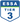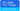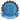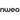# 5 Tricks To Help Improve Your Students' Mental Math Ability

All Posts

As students progress in their academics, their ability to mentally calculate math sums and solve problems improves. From calculating simple addition and subtraction to remembering the square root of integers, mental math comprises of specific learning techniques that help students solve math problems quickly.

Research from The Department Of Education, UK shows that learning key math facts ‘by heart’ enables children to concentrate on the calculation which in turn helps them to develop calculation strategies. Using and applying these strategies to practical use helps them work out answers and remember more facts. (source: The National Strategies; Teaching Children To Calculate Mentally, 2010)

School curriculums often include topics that develop and strengthen students’ mental math calculations throughout the year. Apart from that, teachers also use efficient strategies to build students’ mental math skills and raise their awareness and understanding of the range of mental math methods that they can work on. This also helps in developing their confidence and fluency by practising math problems using these strategies.

To help students improve their mental math proficiency and progressively solve difficult math problems in less time, teachers can implement a range of suitable classroom resources and tricks.

Here are the 5 math tricks to help improve the mental math ability of your students:

## 1. Make It EasyStudents can sometimes find it challenging to multiply or add big denominations. A good strategy is to help them simplify the problem by temporarily shifting the values around.

For example, if the problem is to calculate 791 + 540, it is easier to add 9 to 800 which becomes more manageable to calculate. Now calculate 800 + 540 which is 1340, and take away the additional 9 to get the correct answer 1331.

You can teach students to apply this strategy with multiplication also. For example, if the problem is to calculate 59 x 7, calculate 60 x 7 instead, and then subtract that additional 7, so 420-7 = 413

Calculating with multiples of 10 becomes much easier for students, so always remind them to round off numbers during calculations.This is a very important principle, based on the connection between addition and subtraction. Once this strategy is understood properly, students don’t need to memorise subtraction facts.

For example, if the problem is to find the difference between 14 and 8, instead of subtracting, think “8 plus what makes 14?”  In other words, think of the missing number that has to be added; 8 + ___ = 14. The answer to that is also the answer to 14 − 8.

This principle comes in especially handy with subtractions such as 13 − 7, 17 − 8, 16 − 9, and other basic subtraction facts where the minuend is between 10 and 20. But you can also use it in multitudes of other situations. For example, 72 − 55 is easier to solve by thinking of addition: 55 + 17  makes 72, so the answer to 72 − 55 is 17.

## 3. Tough Multiplications Made Simple

Multiplying large numbers can be challenging for students. So, the most logical thing to teach is how to simplify the numbers and then multiply them. Some cool multiplication tips have been given below that your students can follow:

• The easiest multiplication trick to remember is when multiplying any number by 10 just add a zero to the end of the number. For example, 62 x 10 = 620.
• If one of the numbers is even, you can divide the first number in half, and then double the second number. For example, 20 x 120 can also be solved by dividing 20 by 2 which is 10 and doubling 120 which is 240. Then multiply the two answers; the answer is 10 x 240 = 2400.
• There is also an easy trick to multiply any two-digit number by 11. All you need to do is add the two digits of the multiplicand and insert the answer in the center. For example, to multiply 35 by 11, add the numbers 3 and 5 which is 8 and add it in between the two-digit multiplicand; the answer is 385.

## 4. Division Tricks To Remember

To uncomplicate division problems for your students, you can give them a quick list of key facts they can remember to perform division easily. Here’s a quick way to know when a number can be evenly divided by these certain numbers:

•  A number can be divided by 10 if the number ends in 0
•  A number can be divided by 9 when the digits are added together and the total is evenly divisible by 9
•  A number can be divided by 8 if the last three digits are evenly divisible by 8 or are 000
•  A number can be divided by 6 if it is an even number and when the digits are added together the answer is evenly divisible by 3
•  A number can be divided by 5 if it ends in a 0 or 5
•  A number can be divided by 4 if it ends in 00 or a two-digit number that is evenly divisible by 4

## 5. Solving Percentage ProblemsAs students progress in class, topics like finding the percentage of a number become somewhat tricky but using the right math strategies and tricks can help them tackle these problems with ease.

For example, finding the percentage of 5 for any number can be done within seconds. Follow this method to find what is 5% of 235:

Step 1: Move the decimal point over by one place, 235 becomes 23.5
Step 2: Divide 23.5 by 2 and the answer is 11.75. That is also the answer to the original equation.

Regularly working on the development of your students’ mental math skills not only helps them in improving but also gives them a sense of confidence to solve more math problems. Even if you can’t devote an entire class for mental math, teachers should look out for opportunities to introduce short periods of mental calculations in between lessons and classes to keep their students’ minds fresh and running.

Implementing these 5 useful mental math tricks will surely help your students solve math problems faster as well as make the subject more interesting moving forward.

## Introduce Prodigy In class

You can also try out game-based math platforms that have a greater impact on improving students’ math skills than any other teaching strategies. Prodigy is one such free online math platform specifically designed for students from grades 1-8 to help them master complex math problems while solving puzzles, winning battles and exploring the Prodigy universe.••••